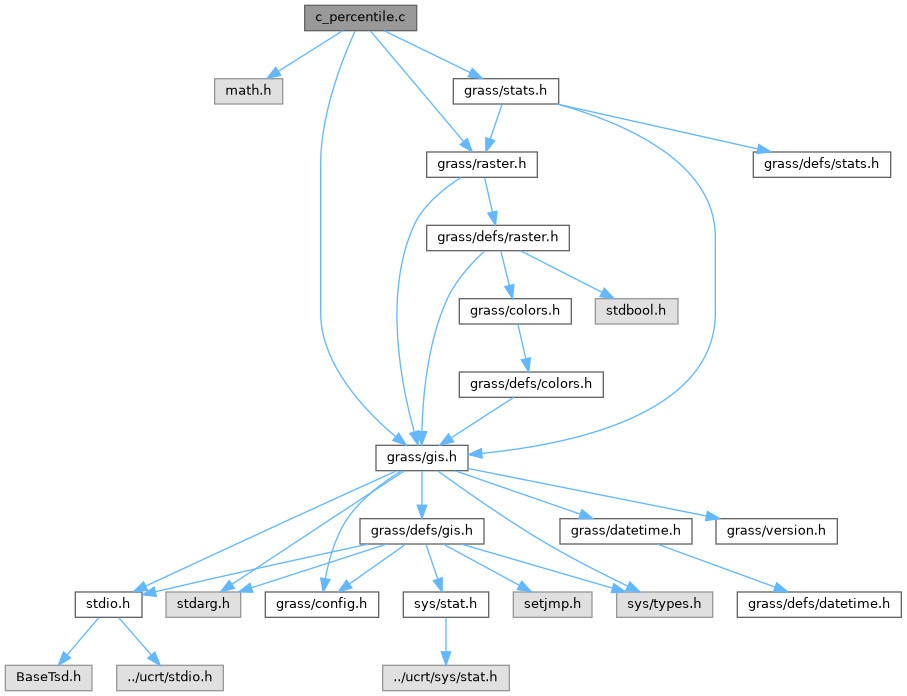GRASS GIS 8 Programmer's Manual  8.2.2dev(2023)-3d2c704037
c_percentile.c File Reference
`#include <math.h>`
`#include <grass/gis.h>`
`#include <grass/raster.h>`
`#include <grass/stats.h>`
Include dependency graph for c_percentile.c:Go to the source code of this file.

## Functions

void c_quant (DCELL *result, DCELL *values, int n, const void *closure)

void c_quart1 (DCELL *result, DCELL *values, int n, const void *closure)

void c_quart3 (DCELL *result, DCELL *values, int n, const void *closure)

void c_perc90 (DCELL *result, DCELL *values, int n, const void *closure)

void w_quant (DCELL *result, DCELL(*values), int n, const void *closure)

void w_quart1 (DCELL *result, DCELL(*values), int n, const void *closure)

void w_quart3 (DCELL *result, DCELL(*values), int n, const void *closure)

void w_perc90 (DCELL *result, DCELL(*values), int n, const void *closure)

## ◆ c_perc90()

 void c_perc90 ( DCELL * result, DCELL * values, int n, const void * closure )

Definition at line 42 of file c_percentile.c.

References c_quant().

## ◆ c_quant()

 void c_quant ( DCELL * result, DCELL * values, int n, const void * closure )

Definition at line 7 of file c_percentile.c.

References Rast_set_d_null_value(), and sort_cell().

Referenced by c_perc90(), c_quart1(), and c_quart3().

## ◆ c_quart1()

 void c_quart1 ( DCELL * result, DCELL * values, int n, const void * closure )

Definition at line 30 of file c_percentile.c.

References c_quant().

## ◆ c_quart3()

 void c_quart3 ( DCELL * result, DCELL * values, int n, const void * closure )

Definition at line 36 of file c_percentile.c.

References c_quant().

## ◆ w_perc90()

 void w_perc90 ( DCELL * result, DCELL(*) values, int n, const void * closure )

Definition at line 88 of file c_percentile.c.

References w_quant().

## ◆ w_quant()

 void w_quant ( DCELL * result, DCELL(*) values, int n, const void * closure )

Definition at line 48 of file c_percentile.c.

References Rast_set_d_null_value(), and sort_cell_w().

Referenced by w_perc90(), w_quart1(), and w_quart3().

## ◆ w_quart1()

 void w_quart1 ( DCELL * result, DCELL(*) values, int n, const void * closure )

Definition at line 76 of file c_percentile.c.

References w_quant().

## ◆ w_quart3()

 void w_quart3 ( DCELL * result, DCELL(*) values, int n, const void * closure )

Definition at line 82 of file c_percentile.c.

References w_quant().